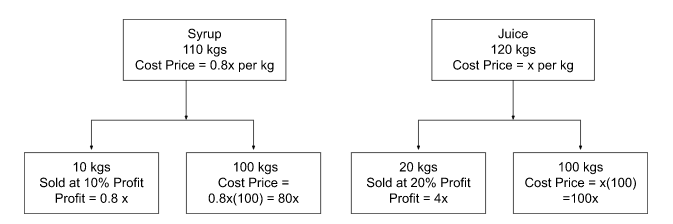# CAT 2022 Question Paper | Quant Slot 1

###### CAT Previous Year Paper | CAT Quant Questions | Question 14

CAT 2022 Quant was dominated by Arithmetic followed by Algebra. In Arithmetic, the questions were dominated by topics like Speed-time-distance, Mixture and Alligations. This year, there was a surprise. The questions from Geometry were relatively on the lower side as compared to the previous years. There were 8 TITA Qs this year. Overall this section was at a medium level of difficulty.

Question 14 : Amal buys 110 kg of syrup and 120 kg of juice, syrup being 20% less costly than juice, per kg. He sells 10 kg of syrup at 10% profit and 20 kg of juice at 20% profit. Mixing the remaining juice and syrup, Amal sells the mixture at ₹ 308.32 per kg and makes an overall profit of 64%. Then, Amal's cost price for syrup, in rupees per kg, is

## Best CAT Coaching in Chennai

#### CAT Coaching in Chennai - CAT 2022Limited Seats Available - Register Now!

Let the Cost Price of Juice be rupees x per kg.
Since the Cost Price of Syrup is 20% less than the Cost Price of Juice, Cost Price of Syrup is 0.8x per kg.
Total Cost Price of Syrup = 110 * 0.8 x = 88 x
Total Coat Price of Juice = 120 * x = 120x
Total Cost Price = 88x + 120x = 208x
Since the Overall Profit Percentage is 64%.
Total Profit = 0.64(208x) = 133.12xThe profit generated on selling 10 kgs of Syrup which costs 0.8x rupees per kg at 10% Profit = 0.1 * 10 * 0.8x = 0.8x
The profit generated on selling 20 kgs of Juice which costs x rupees per kg at 20% Profit = 0.2 * 20 * x = 4x
The remaining profit (133.12x - (0.8x + 4x) = 128.32x) is generated by selling 100 kgs of Syrup and 100 kgs of Juice at ₹ 308.32 per kg.
The total Selling Price of 100 kgs of Syrup and 100 kgs of Juice is 200 * 308.32 = 2(30832)
Cost Price of 100 kgs of Syrup = 0.8x * 100 = 80x
Cost Price of 100 kgs of Juice = x * 100 = 100x
Total Cost Price = 80x + 100x = 180x
Profit = Selling Price - Cost Price
128.32x = 2(30832) - 180x
308.32x = 2(30832)
x = 200
Cost Price of Syrup per kg = 0.8 x = 0.8(200) = ₹160 per kg.

###### CAT Coaching in ChennaiCAT 2023

Classroom Batches Starting Now! @Gopalapuram

###### Best CAT Coaching in Chennai Introductory offer of 5000/-

Attend a Demo Class

##### Where is 2IIM located?

2IIM Online CAT Coaching
A Fermat Education Initiative,
58/16, Indira Gandhi Street,
Kaveri Rangan Nagar, Saligramam, Chennai 600 093

##### How to reach 2IIM?

Mobile: (91) 99626 48484 / 94459 38484
WhatsApp: WhatsApp Now
Email: info@2iim.com